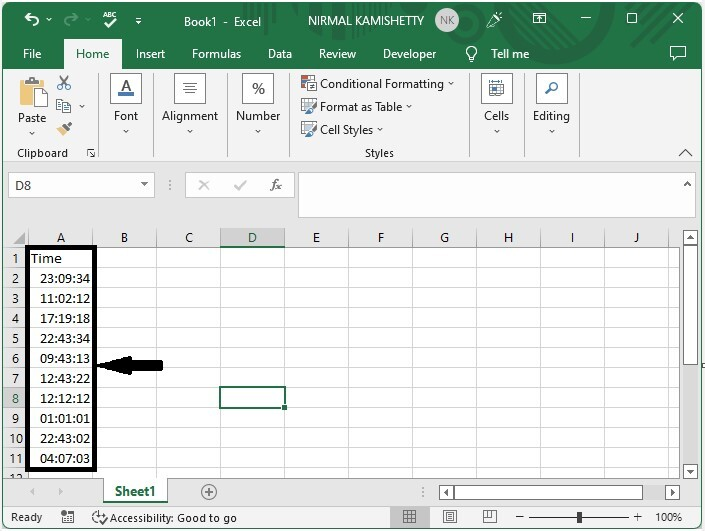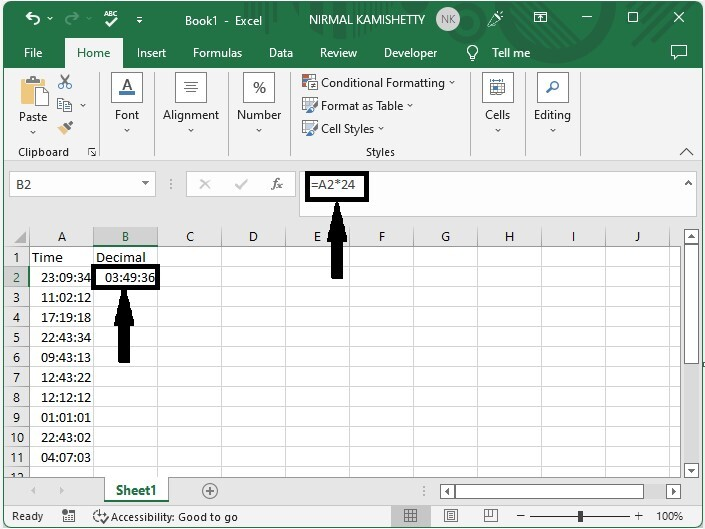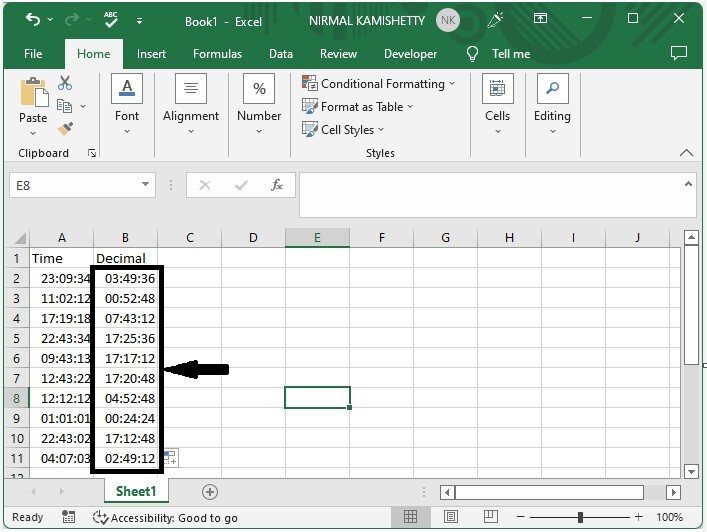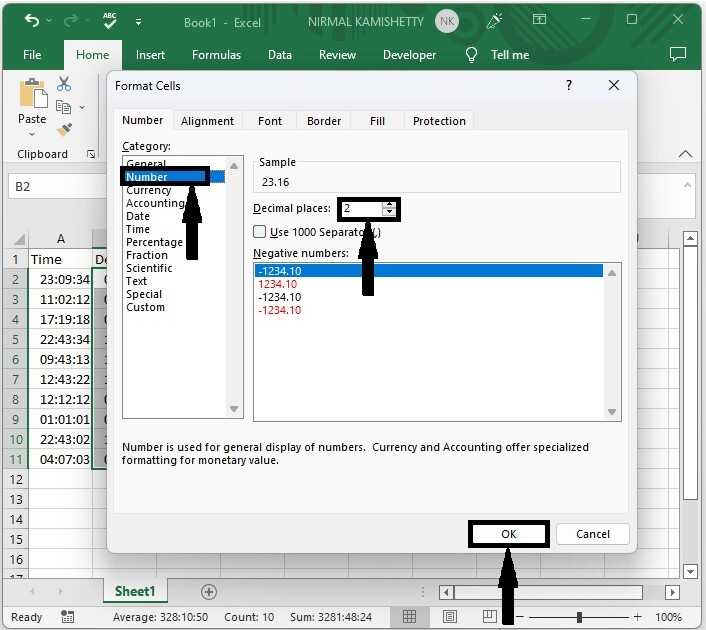# How to Convert Time to Decimal Hours /Minutes/ Seconds in Excel?

A decimal hour is a time that is represented as a number. In Excel, you may have needed to convert the given time to decimal values at times. If we try to do this task manually, then it can be a time-consuming process. We can use the faster approach mentioned in this article. We can solve this task using the formulas supported in Excel. Read this tutorial to learn how you can convert time to decimal time in Excel.

## Converting Time to Decimal Hours in Excel

Here we will use the formula to get any one of the values, then use the auto-fill handle to fill all values, and use the Format function to complete the task. Let's go over a simple procedure for converting time to decimal time in Excel using formulas.

### Step 1

Consider an Excel sheet with data in the form of a list of times, as shown in the image below.Now click on an empty cell, in our case cell B2, and enter the formula as =A2*24 and click enter to get our first result, as shown in the below image.

Empty cell > Formula > Enter### Step 2

Then drag down the first result using the auto-fill handle to fill all the values as shown in the below image.### Step 3

Now we need to convert those values to decimals. To do that, select the data, then right-click and select format cells. Then click on the number, change the decimal to two, and click as shown in the below image to complete our process.

Select data > Right click > Format cells > Number > Two > OKIf we need to convert the time to decimal minutes, we can use the formula =A1*24*60.

To convert time to decimal seconds, use the formula =A1*24*60*60.

## Conclusion

In this tutorial, we used a simple example to demonstrate how you can convert time to decimal hours, minutes, and seconds in Excel.

Updated on: 06-Mar-2023

30K+ Views